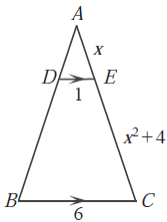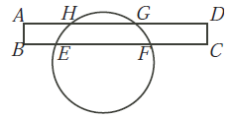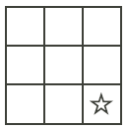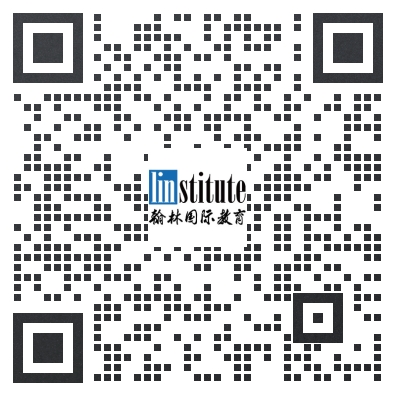# 2008COMC加拿大数学公开赛真题免费下载

### 真题与答案下载### 2008 COMC真题免费下载

Part A共8题，每题5分

Part B共4题，每题10分

#### 部分真题预览：

Part A :

4)In the diagram, D is on AB and E is on AC with DE parallel to BC. Also, DE = 1, BC = 6, AE = x, and EC = x2 + 4. Determine all possible values of x.6)Rectangle ABCD intersects a circle at points E, F,G, and H, as shown. If AH = 4, HG = 5 and BE = 3, determine the length of EF.7)A star is placed in the bottom right corner square of a 3 × 3 grid, as shown. A fair coin is flipped repeatedly. Each time that the coin shows heads, the star is moved one space upwards; each time that the coin shows tails, the star is moved one space to the left. (The star may move off the grid.) Determine the probability that the star reaches the top left corner square of the grid.Part B :

4)A triangle is called automedian if its three medians can be used to form a triangle that is similar to the original triangle.

1. Show that the triangle with sides of length 7, 13 and 17 is automedian.
2. ΔABC has side lengths AB = c, AC = b and BC = a, with a < b < c. If ΔABC is automedian, prove that a2 + c2 = 2b2.
3. Determine, with proof, an infinite family of automedian triangles with integer side lengths, such that no two of the triangles in the family are similar.

### 2008 COMC加拿大数学奥赛完整版答案免费下载